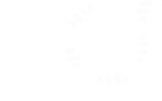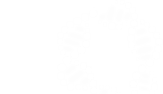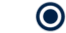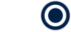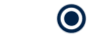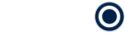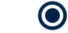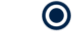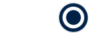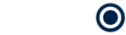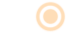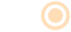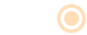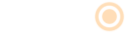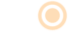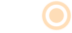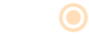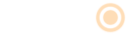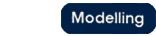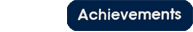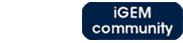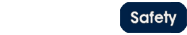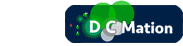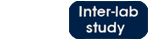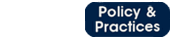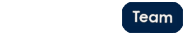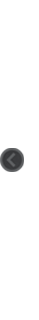# Team:Oxford/how much can we degrade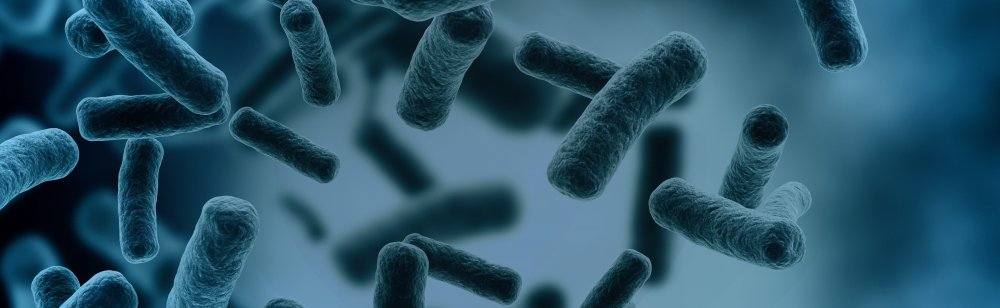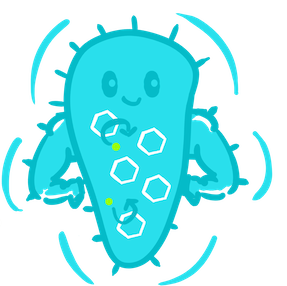# Introduction

Before we began using synthetic biology to develop a system for bioremediation of chlorinated waste, we thought it was important to work towards an answer to the above question. To do this, we used information from the literature (Gisi et al, 1998) about the metabolism of the native bacterium Methylobacterium extorquens DM4.

We then worked on a model to calculate both the pH change of the system and the volume of DCM degraded over time. This was achieved by using a combination of Michaelis-Menten kinetics, ordinary differential equations and stoichiometric relations.

How much DCM could the native bacterium degrade?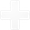How much DCM could the native bacterium degrade?
Calculating total DCM degraded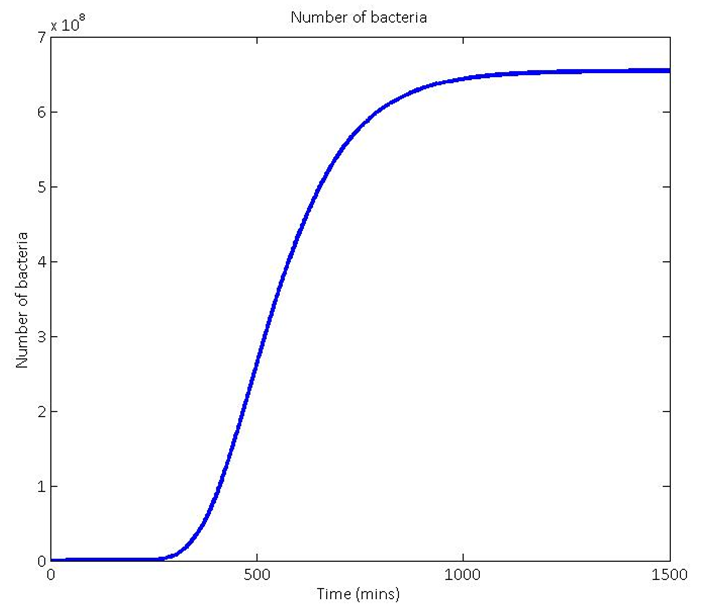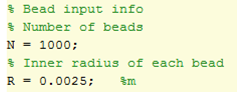# 1) Obtaining a theoretical growth curve

To start this calculation, we needed to know how many bacteria we could expect to have in our system. To do this, we used the realistic bead dimensions and numbers shown in the Matlab screen shot on the left. This allowed us to calculate the volume of bacteria we predict to be infused the agarose beads. We then used the assumption that the bacteria would grow to an optimum density of 10^7 bacteria per ml of agarose and combined these to give us an approximation of how to scale the growth curve: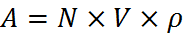• A = Gompertz vertical scaling constant
• N = number of beads
• V = volume of each bead in ml
• ρ = number of bacteria per ml

• Our theoretical growth curves were based on Gompertz functions for the reasons explained when you follow this link: (what are Gompertz functions?). An example output growth curve of the model is shown here.

The scaling of the growth rate of the Gompertz function comes directly from growth curves of the DM4 bacteria that we obtained in the lab. See more about our work with growth curves here.

# 2) Calculating the volume of DCM that the bacteria can degrade

Our next task was to model the average rate of DCM degradation by M. extorquens DM4. Using Michaelis-Menten kinetics, this was predicted to be: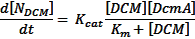• d[Ndcm]/dt = rate of DCM molecule degradation (s-1)
• kcat = dcmA turnover rate (= 0.6 s-1 for DM4)
• [DCM] = DCM concentration (= 0.02M for our system)
• [DcmA] = Number of DcmA molecules per cell (87576) Where did this number come from?
• Km = Michaelis constant ( = 9 x 10^-6 M for this reaction)

• Through the use of diffusion-limiting beads, [DCM] is kept constant at 0.02M. This is significantly larger than our Michaelis constant, so this equation can be simplified by using the following assumptions: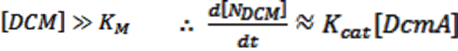Multiplying this by our population function, the total rate of DCM degradation is given as: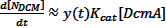Turning this into a more recognisable value (a volume) gives the total rate of DCM degradation as: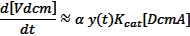Where: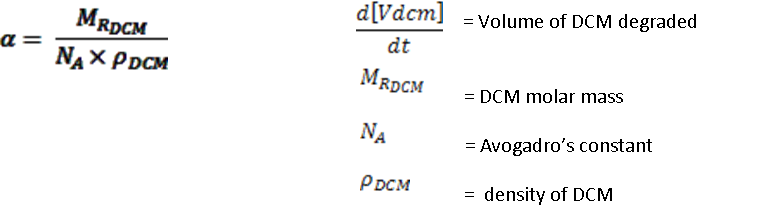When all of these calculations were modelled in Matlab with the input conditions shown above, the total volume of DCM that we would predict the native bacteria DM4 to degrade in 24 hours is shown by the red line below. This is before you account for the possible toxicity of the pH drop. This is taken into account in the sections below.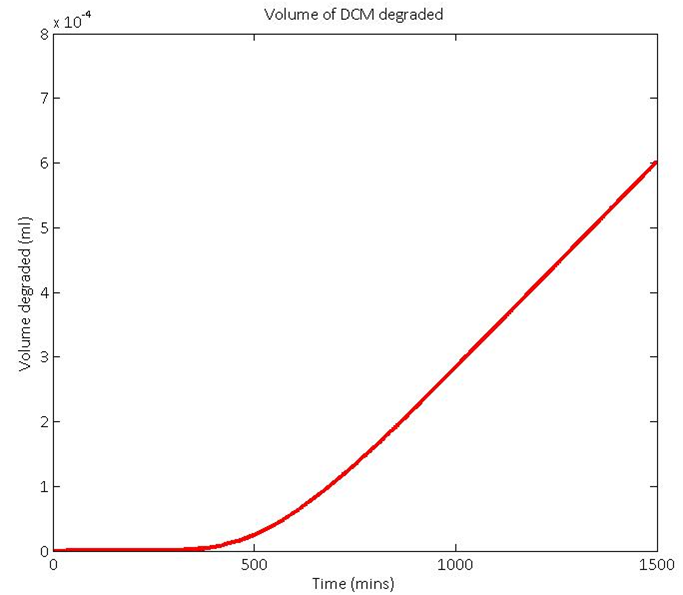# References:

1. (Dr George Wadhams, personal communication, August 4, 2014)
2. Michaelis L. and Menten M.L. Kinetik der Invertinwirkung Biochem. Z. 1913; 49:333–369 English translation Accessed 6 April 2007

How much would the pH change by?How much would the pH change by?
Calculating the pH change

The degradation of DCM by DcmA produces hydrochloric acid (HCl) according to the reaction below: ￼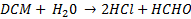There is a resulting pH change because of the accumulation of HCl. Because we are dealing with an organic system which cannot tolerate pH<6, we must track the anticipated HCl production and resulting pH change. The following relationships were used: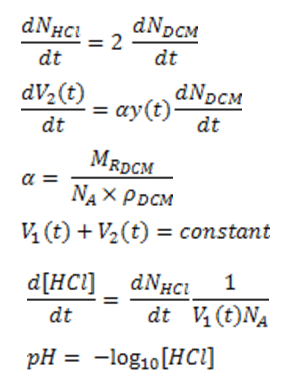• NHCL = molecules of HCl
• NDCM = molecules of DCM
• V1(t) = aqueous layer volume (ml)
• V2(t) = DCM layer volume (ml)
• y(t) = bacteria population
• MRDCM = DCM molar mass
• NA = Avogadro’s constant (mol-1)
• ρDCM = DCM density (kg/dm3)
• [HCl] = HCl concentration (M)

• These equations were then simulated using a series of linked functions on MatLab and the results are displayed below: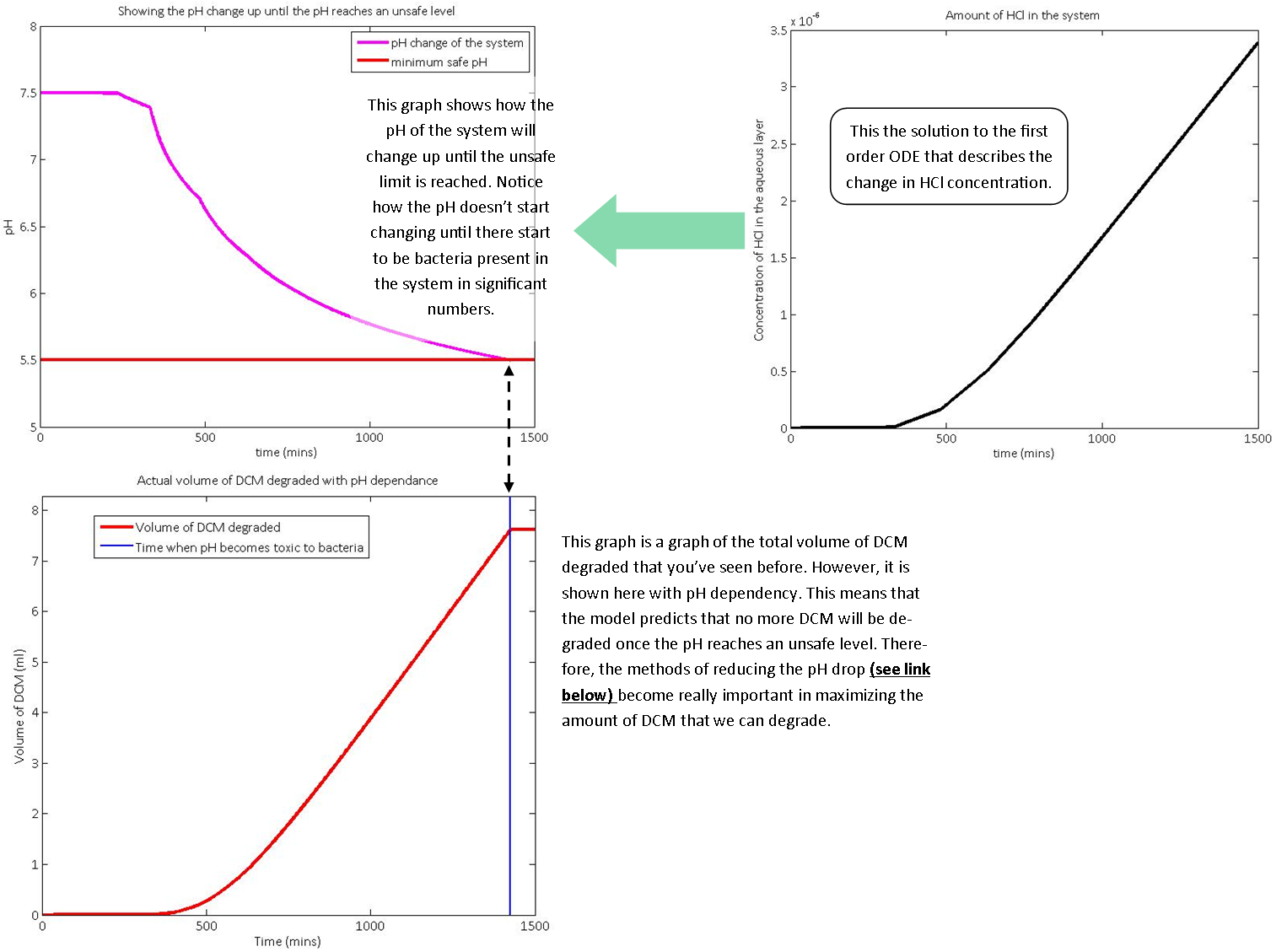# Summary:

As you can see from the above graph, the native bacterium M. extorquens DM4 will not be able to degrade a large volume of DCM. It will therefore not be a suitable to dispose of chlorinated waste efficiently. There are several reasons for this, including:

• The degradation of DCM is a stress response for M. extorquens DM4. Therefore, when metabolising DCM, it is also up-regulating stress response molecules such as repair enzymes, which is an additional strain on cellular metabolism.
• M. extorquens DM4 has a doubling rate of 8-9 hours, so it takes 2 weeks to grow up a colony. Additionally, they proved very difficult to grow in the lab, both on standard growth agars and specialised nutrient agars.
• M. extorquens DM4 are not yet well-understood bacteria, particularly with respect to their metabolism.

• However, using synthetic biology, we can dramatically increase the amount of chlorinated solvents that certain bacteria can degrade. This is because:

• We will use E. coli and P. putida in order to break down DCM. The advantage is that these are extremely well-characterised bacteria that are easy to grow in the lab.
• We are expressing microcompartments in both E. coli and P. putida, which prevent toxic intermediates of DCM metabolism from damaging the cells. This is necessary because unlike M. extorquens DM4, E. coli and P. putida have not evolved for the degradation of DCM and toxic intermediates released during its metabolism
• We will upregulate and express formaldehyde dehydrogenase in P. putida and E. coli, respectively. This will help the cells deal with formaldehyde, which is a genotoxic intermediate produced in the degradation of DCM.

• This model proves the power of computer modelling and shows the importance of using synthetic biology to solve global problems. The exact amount of DCM that could be degraded depends largely on input conditions, such as the number of beads. While more beads in the system allow more rapid DCM removal, a very large system can provide challenging to construct and monitor. (What do we mean by beads?)
What is a Gompertz function?What is a Gompertz function?
Gompertz Functions

We used a variation of a sigmoid function called a Gompertz function to model the theoretical growth of our bead-encapsulated bacteria. These functions are well-established as a method of predicting population growth in a confined space, which will be the case if we encapsulate them in agarose beads. Growth rates follow a sigmoidal curve, where they first increase and then slow because of limited resources and population density. We assumed that the population of bacteria over time will follow one of these functions (when scaled correctly).

Gompertz functions are of the form: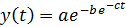• y(t) = population size as a function of time
• A = maximum sustainable population
• B = shift on time axis
• C = growth rate

• Using this theoretical form, we could then calibrate the values of our variables through comparison with actual growth curve data from wet lab experiments. This was an important step because it then allowed us to calculate the total theoretical degradation rate of DCM that our kit can support.

Varying each of the three constants allows us to fit our Gompertz function to the actual growth data. The effect of varying each constant is shown below: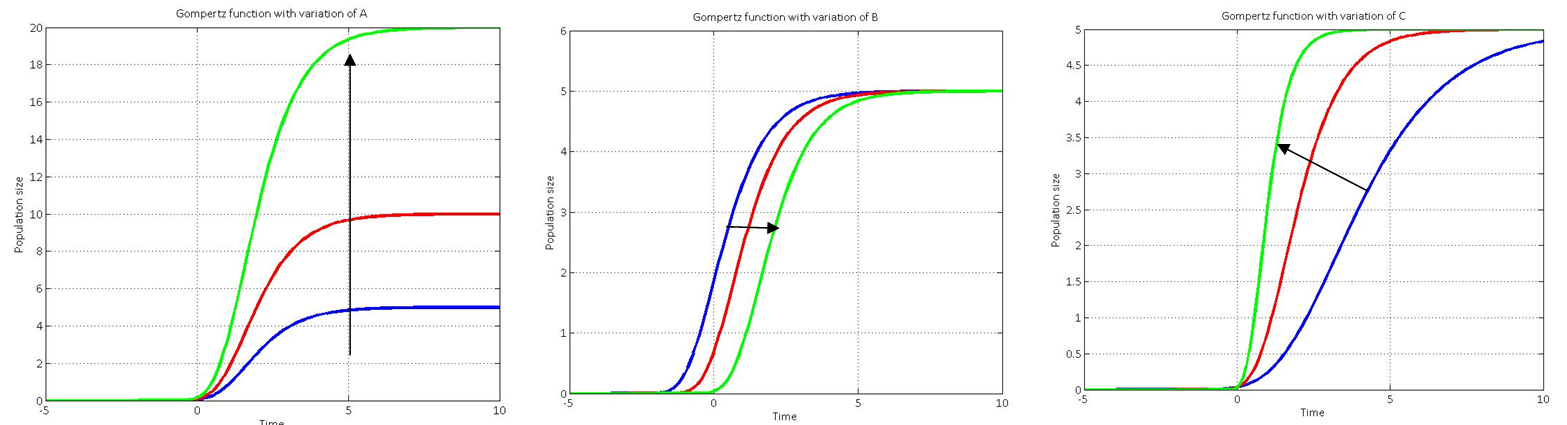# Reference:

Zwietering, M. H.; Jongenburger, I.; Rombout, F. M.; van 't Riet, K. (1990), "Modeling of the Bacterial Growth Curve", Applied and Environmental Microbiology 56 (6): 1875–1881
How can we reduce the drop in pH?How can we reduce the drop in pH?
Using buffers to reduce the pH change of our system

We have investigated the effect of using buffers in the aqueous part of our system.
As a first approximation, we model our system of bacteria turning over DCM, producing HCl, as a chemical system in which HCl immediately enters the 'bulk' (extracellular) solution; in this system we have a single buffer (HEPES) to reduce the drop in pH, maximising the amount of DCM the entire system can degrade before the pH drops below a toxic level.

Derivation of the Van Slyke equation:

To simplify calculations, assumptions that HCl completely dissociates, and that the system volume = 1L (allowing concentration and number of moles to be treated interchangeably) are made.

Electro-neutrality condition for a system of two substances, HA and BOH:

# (1.1)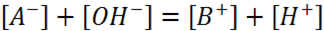Total concentration of buffer:

# (1.2)By definition:

# (1.3)Combining (1.2) and (1.3):

# (1.4)Combining this with (1.1) and the water auto-ionisation constant definition, K_W=[H^+ ][〖OH〗^-], give the moles of strong acid added:

# (1.5)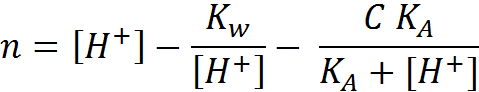Differentiating with respect to the pH gives the buffer capacity:

# (1.6)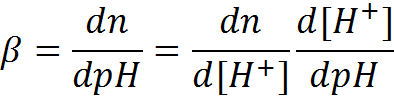# (1.7)# (1.8)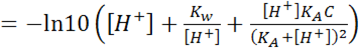Which can be generalized for multi-buffer systems:

# (1.9) Van Slyke equation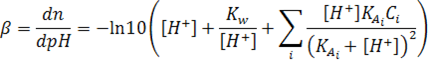• β = buffer capacity
• n = number of equivalents of strong acid added (per L solution) – we have this as a function of t: approximately addition at a constant rate.
• K_(A_i) = K_A of component buffer i
• K_W = ionic product of water, 10^(-14)
• C_i = concentration of component buffer i

• Taking the reciprocal, and substituting the definition:

# (1.10)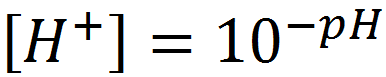gives:

# (1.11)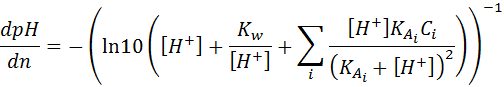# (1.12)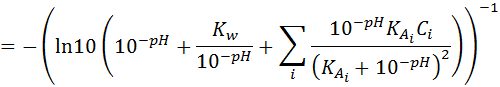For a single buffer system: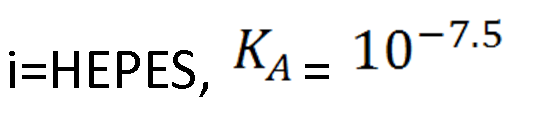Numerically solving this ODE in MATLAB, for pH(n, C) and hence pH(t, C) gives: Upon solving the equation in Matlab, it was clear that only a relatively low concentration (0.05 M) of buffer was needed to significantly reduce the pH change of the solution: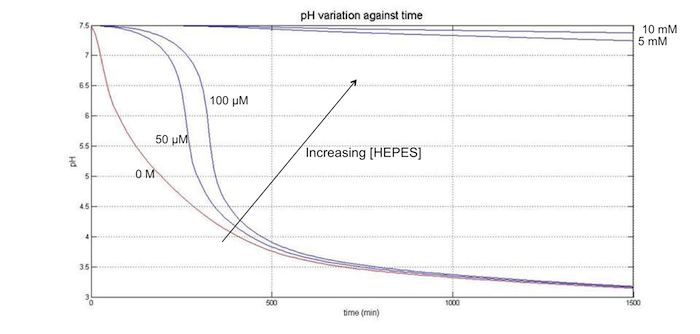The numerical solution to this differential equation was confirmed by reducing the n interval by a factor of 100, which gave the same result.

Derivation shown is based on Adam Hulanicki book Reakcje kwasów i zasad w chemii analitycznej, 2nd ed., PWN, Warszawa 1980 (English edition: Reactions of acids and bases in analytical chemistry; Chichester, West Sussex, England: E. Horwood; New York: Halsted Press, 1987).

Another possibility of reducing the overall pH change is adding a lot more water to the system. This is the easier method and could be used for single-use DCM disposal kits. However, it is impractical in large scale applications because of the very large amount of water that would have to be added.
How does the amount of water added affect the output?How does the amount of water added affect the output?
Calculating the pH change

We then used our model to predict the effect on the system if you simply increase the amount of water in the aqueous layer. This shows how much water is necessary to prevent the pH from dropping too much. It demonstrates why addition of a buffer is the more reasonable choice to control the pH of the system.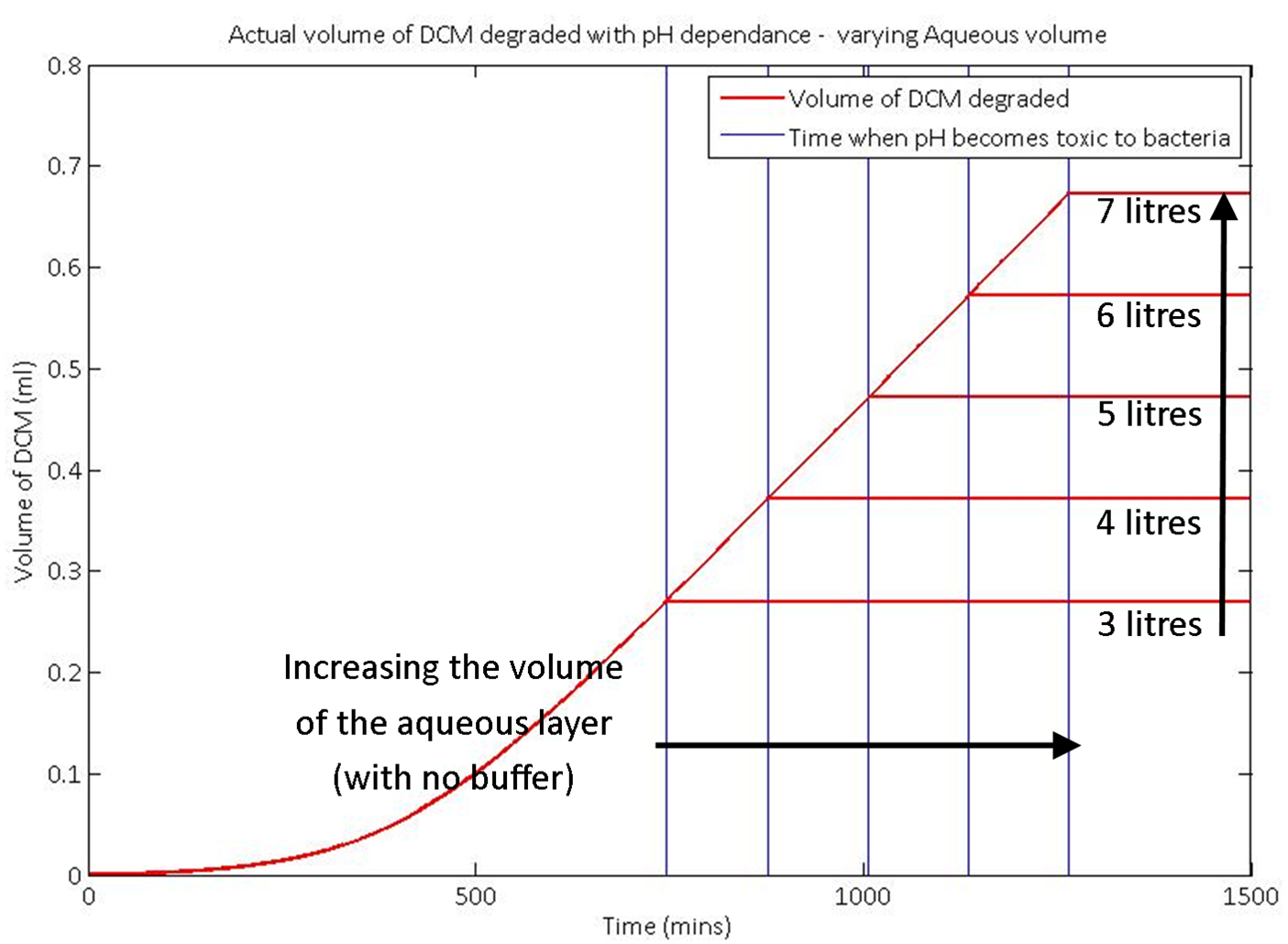The graph here is for non specific inputs and is for demonstration purposes only. It shows well how the model responds to changing the input values.

Buffers?
How does the kcat of the system affect the output?How does the kcat of the system affect the output?
The apparent uni-molecular rate constant kcat, also called the turnover number, denotes the maximum number of enzymatic reactions catalysed per second.

We used our model to predict the response of the system to a change in the kcat value of the DCM degradation enzyme, dcmA. Increasing the value of kcat by a significant amount is unrealistic in the length of our project. However, in future work, the kcat could potentially be substantially improved.

In the graph shown here, the total volume degraded doesn't change. This is because the amount of HCl that the system requires to reach a toxic pH level is constant, as we are not varying the volume of the aqueous layer. To increase the total amount of DCM degraded, we simply need to add more water or a pH buffer to the system. However, increasing the kcat value dramatically increases the rate of the degradation. This hints towards a valid future area of research.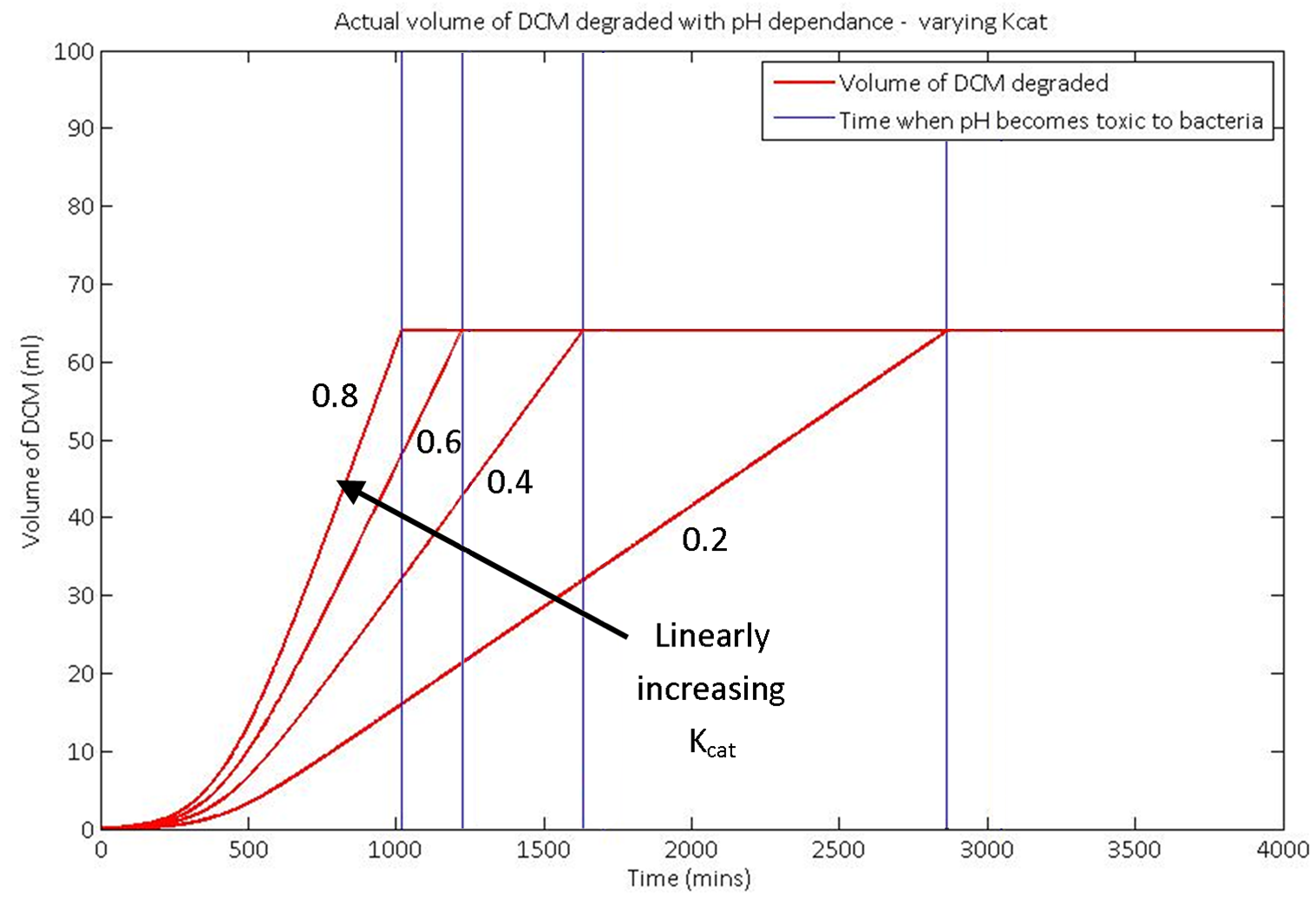The graph here is for non specific inputs and is for demonstration purposes only. It shows well how the model responds to changing the input values.
Potential benefits?Potential benefits?
Increasing the kcat of the enzyme greatly improve our system, as you can see in the models shown above.

By simple adjustment of input parameters, our model could be adapted to simulate the degradation of other types of toxic compounds in other bacteria with different enzymes. This modelling technique is therefore particularly powerful, because if you know certain parameters about the system, you can simulate how much of a particular product can be produced by a bacterial system.

More broadly, the potential benefit of months of synthetic biology research could be analysed within a few hours using this model, as long as the relevant parameters are roughly known.

To demonstrate what we mean by this, here are some other processes with different kcat values: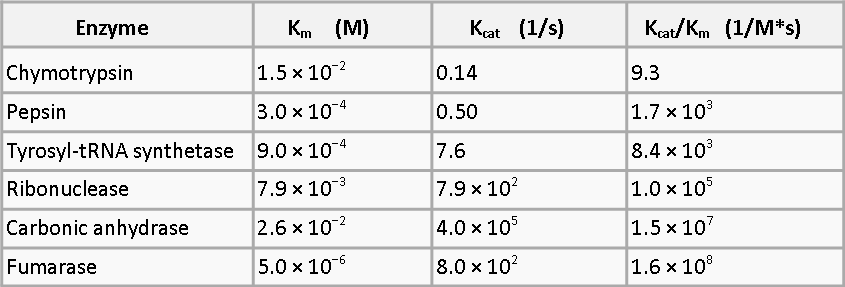As you can see, using systems with the parameters shown above would increase the amount of product obtained from the same number of bacteria by orders of magnitude and would therefore be highly beneficial to a bioremediation system. Future work could definitely involve modelling these reactions and investigating the potential benefits before the wet lab work begins.

# Reference:

1.  Mathews, C.K.; van Holde, K.E.; Ahern, K.G. (10 Dec 1999). Biochemistry (3 ed.). Prentice Hall. ISBN 978-0-8053-3066-3.
How can we use the pH drop?How can we use the pH drop?
How could we measure the pH?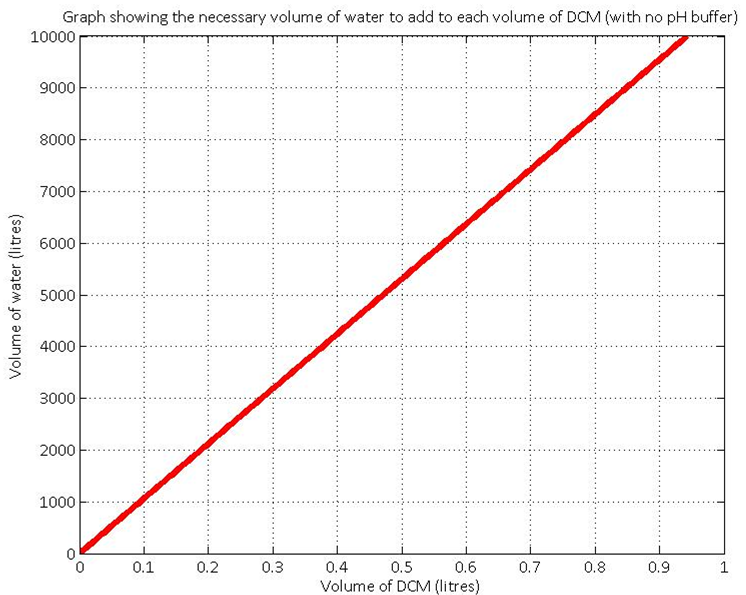As we’ve built the model predicting the pH change very accurately, we have been thinking about how to use this system change to our advantage. There are two viable options that we’ve considered.

By using a pH indicator that changes colour at a pH of around 6, we could use the same electronics that we’ve developed for detecting the fluorescence of the sfGFP in the biosensor to detect the colour change, and therefore the point at which the pH becomes dangerously low. This has the advantage of making the biosensor very user friendly while keeping the system cheap. The other option is to use a commercially available digital pH meter to signal a warning when the pH gets too low. This could require occasional maintenance of the pH sensor, but would have the advantage of being more accurate.

How is the pH useful?

The pH in our system is an indirect measure of the amount of DCM that we’ve degraded. It is therefore possible to calculate the required amount of water that has to be added to a certain amount of DCM to ensure the pH remains neutral. If no buffer solution is added, initial calculations (see the graph) indicate that there is a very big difference between the relative volumes of the amount of DCM added and the volume of the aqueous layer. This highlights the importance of using a pH buffer solution in the aqueous layer.

Therefore, the system that detects the amount of DCM that we’ve degraded could link the digital pH read-out to the initial amount of water added.